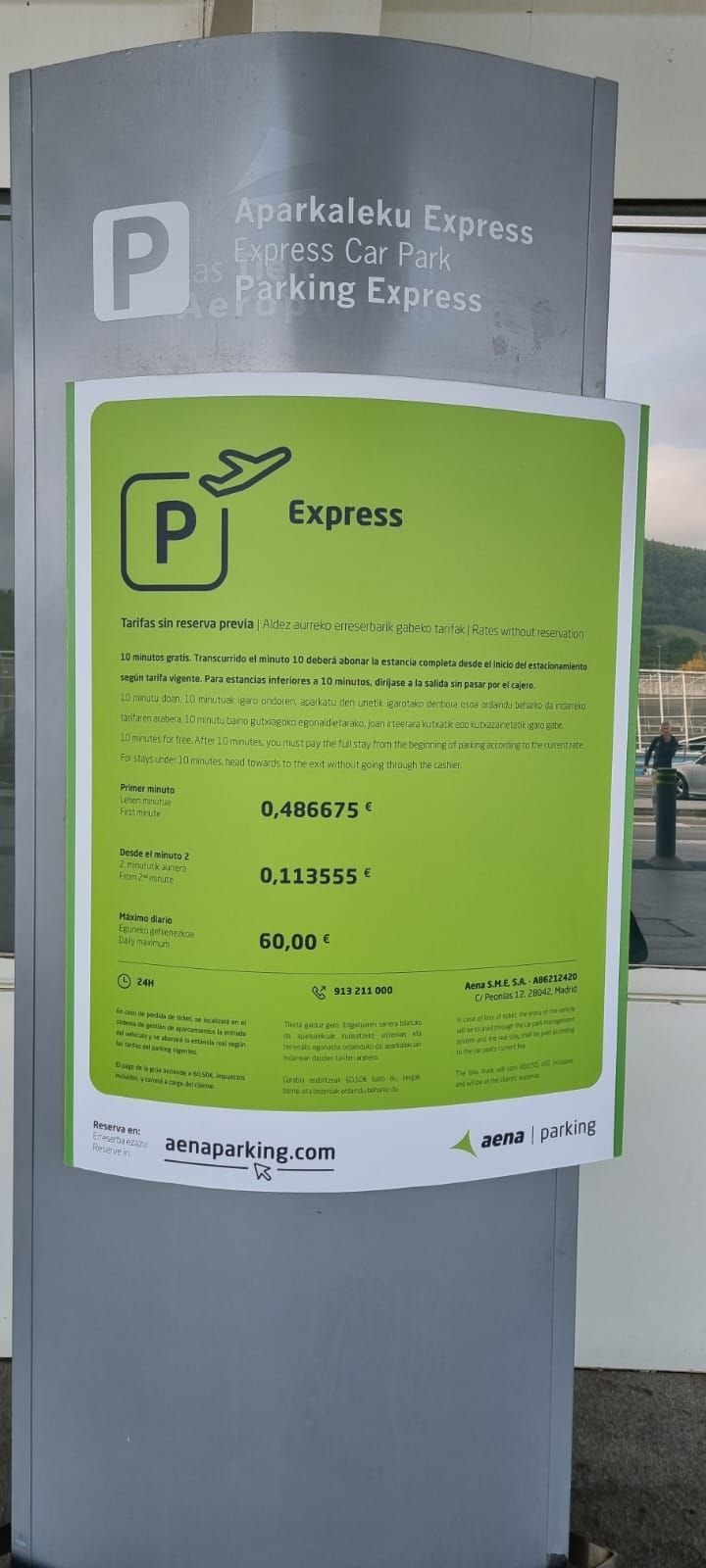Chris Sangwin pointed me at the following photo:… with the comment that that was an awful lot of significant figures. It’s hard to fathom why a car park would give its prices to the nearest semimillieurocent, honestly, but presumably there’s a reason behind it.

So, the first minute’s parking costs €0.486675, and every further minute costs €0.113555. Why those numbers?

Some observations:

• The ratio between the numbers is very close to 30:7.
• Two hours costs €14, almost exactly.
• Eight and three-quarter hours costs €59.99, just less than the daily rate.
• Ten minutes costs €1.51.

None of those numbers are bang-on perfect. Perhaps there’s a rounding error?

### An exploration

Suppose we want to charge €14 for two hours and €60 for 8h45, with the same structure – a price, €$f$, for the first minute and a (probably) different price €$s$, for each subsequent minute.

Then we’ve got two simultaneous equations:

• $f + 119p = 14$
• $f + 524p = 60$

That leads to $405p = 46$, and $p\approx 0.11358$, fully two and a half millicents more than Aena charge. ($f$, for the record, would be around 0.48395, about 2.5 millicents less.)

In my opinion, that’s close but no cigar. If they’re going to give prices to six decimal places, they should work to six decimal places.

### A possibility

One thing I noticed is that the original numbers are very close to $f=\frac{420}{863}$ and $s=\frac{98}{863}$ – indeed, this gives exactly €14 for two hours. However, there are infinitely many combinations of numbers that satisfy that. Why those?

We want another solution to $f + Mp = T$, where $M$ (minutes) and $T$ (total price) are positive integers. The next one after $(119, 14)$ appears to be $(863, 98)$ - so parking for 14h24 would theoretically cost €98 (although that’s well beyond the day rate). Using cents rather than euros doesn’t help any.

So, all in all, I’m a bit stumped. Why does a Basque airport car park charge (apparently) in 863rds of a euro? Any ideas? Let me know!

* Thanks to Chris Sangwin for the picture, Tom Briggs for discussing, and Talking Maths in Public for putting up with it.